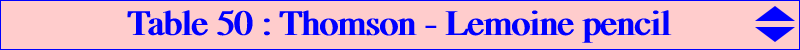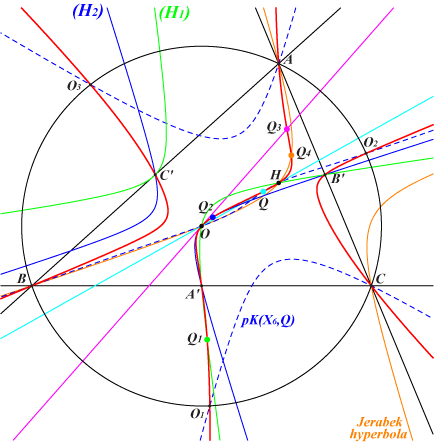The Thomson cubic K002 and the Lemoine cubic K009 are both circum-cubics passing through the vertices of the medial triangle, X(3) and X(4). The tangent at X(3) passes through X(64) except for K009 which has a node at X(3). They generate a pencil of cubics K(Q) through these same 8 points and meeting the Euler line again at Q. All these cubics are psK(Ω, X2, X3) with pseudo-pole Ω on the line X(6)-X(25) or, equivalently, psK(X6 x Q, X2, X3) where X6 x Q is a barycentric product. There is only one pK namely the Thomson cubic. See Pseudo-Pivotal Cubics and Poristic Triangles for further details and CL068 for other psK(Ω, X2, X3). See below for geometric properties of these cubics. The following table shows a selection of these cubics according to the point Q on the Euler line, and also their isogonal transforms..Q Ω K(Q) centers on the cubic : X3, X4, Q and K(Q)* remark on K(Q) X2 X6 K002 X1, X6, X9, X57, X223, X282, X1073, X1249 K002 pK X3 X184 K009 X32, X56, X1147 K028 nodal cubic X4 X25 K376 X64 K443 X5 X51 K026 X5403, X5404 K361 stelloid X20 X154 K426 X3532 K841 central cubic X25 X1974 X206 X30 X1495 K446 X74, X133, X1511 K447 circular cubic X140 X13366 K569 X54, X1493 K849 X235 X2207, X2883 X297 X232 X1987 X403 X113 X426 X1092 X427 X1843 X66, X141, X3162 X429 X960 X439 X3053 X440 X71 X441 X248 X442 X10, X65, X942 X443 X2213 X468 X1177 X858 X2393 X67 X1312 X2575 X1313 X2574 X1368 X69 X1513 X511 X1529 X1503 X1532 X517, X1145, X1457 X1536 X516 X1551 X542 X1567 X2782 X1594 X1209 X2072 X265 X3079 X154 X3150 X879 X3154 X523 X6656 X1194 X695The pencil contains seven other nodal cubics : • three are decomposed into a sideline of ABC and a conic passing through X(3), X(4) and the remaining vertices of ABC and the medial triangle. • four (not always real) with complicated corresponding points Q on the Euler line. ***Properties of K = psK(X6 x Q, X2, X3) with Q on the Euler lineThe cubic K = psK(X6 x Q, X2, X3) meets : • the circumcircle again at the same points O1, O2, O3 as pK(X6, Q), a member of the Euler pencil, the remaining common points being obviously X(3), X(4) and Q. • the line at infinity at the same points as pK(X6, aQ), another member of the Euler pencil, where aQ denotes the anticomplement of Q. The remaining common points lie on the Jerabek hyperbola namely A, B, C, X(3), X(4) and the isogonal conjugate Q4 of aQ. K also passes through : • Q1 = G-Ceva(X4 x Q), on the complement (H1) of the rectangular circum-hyperbola passing through X(20). • Q2 = G-Ceva(X3 x Q), on the complement (H2) of the Jerabek hyperbola. Q2 is the tangential of Q. • Q3 = X1073 x Q, on the line X(3)-X(64), the tangent at X(3) to K. Q3 is therefore the tangential of X(3). Note the triads of collinear points on K : X(3), Q2, Q4 - X(4), Q1, Q4 - Q, Q1, Q3.   See also Table 54 for a generalization.